# 深度生成模型

## 结构化生成模型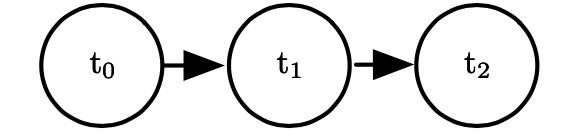## 生成模型涉及的计算

### 配分函数

$Z=\int p(x)\mathrm dx$

# 无向图生成模型

## Boltzmann 机

$p(\boldsymbol v,\boldsymbol h)=\frac1Z\exp\left(-\boldsymbol{v}^{\top} \boldsymbol{R} \boldsymbol{v}-\boldsymbol{v}^{\top} \boldsymbol{W} \boldsymbol{h}-\boldsymbol{h}^{\top} \boldsymbol{S} \boldsymbol{h}-\boldsymbol{b}^{\top} \boldsymbol{v}-\boldsymbol{c}^{\top} \boldsymbol{h}\right)$

Boltzmann 机虽然形式非常简单（仅仅是一个多元正态分布），但由于潜变量的存在，对潜变量求完期望后可以得到任意复杂的 $p(v)$ 的形式，可以证明它可以以任何精度近似离散变量上的概率分布函数。

Boltzmann 机具有难以处理的配分函数，需要用上一节中提到的方法来近似；但或许更严重的问题是不容易进行边缘概率计算，这是因为 $v$$h$ 以复杂的方式纠缠着。基于此，我们可以提出一个受限 Boltzmann 机，它的函数形式是

$p(\boldsymbol v,\boldsymbol h)=\frac1Z\exp\left(-\boldsymbol{v}^{\top} \boldsymbol{W} \boldsymbol{h}-\boldsymbol{b}^{\top} \boldsymbol{v}-\boldsymbol{c}^{\top} \boldsymbol{h}\right)$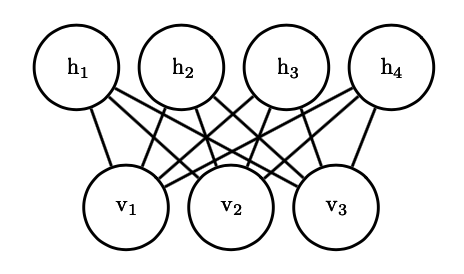$P(\boldsymbol{h} \mid \boldsymbol{v})=\prod_{j=1}^{n_{h}} \sigma\left((2 \boldsymbol{h}-1) \odot\left(\boldsymbol{c}+\boldsymbol{W}^{\top} \boldsymbol{v}\right)\right)$

## 深度 Boltzmann 机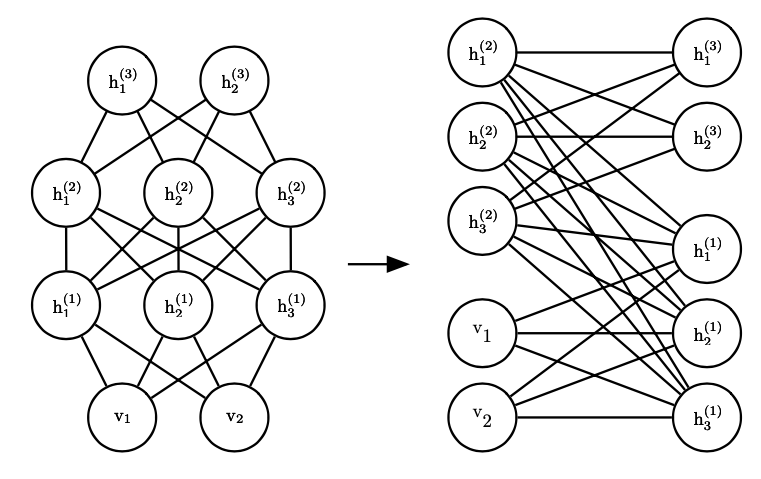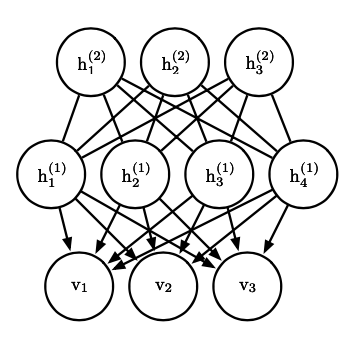# 有向图生成模型

## 自回归网络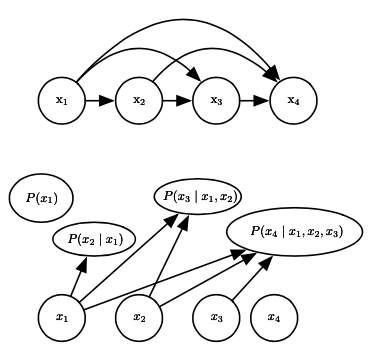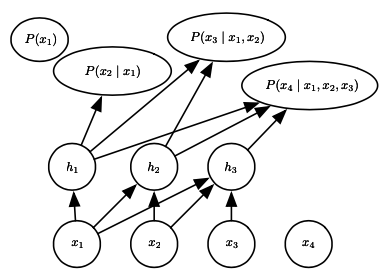## 生成器网络

$p_{z}(\boldsymbol{z})=p(g(\boldsymbol{z}))\left|\operatorname{det}\left(\frac{\partial g}{\partial \boldsymbol{z}}\right)\right|$

$L(q)=\mathbb E_{x\sim q(z|x)}\log p(z,x)+H(q(z|x))$